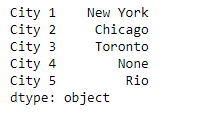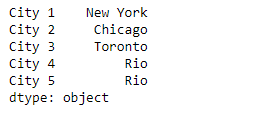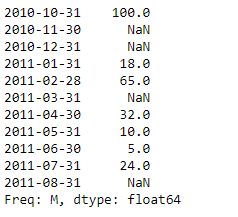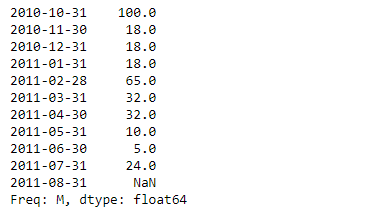# Python | Pandas Series.bfill()

• Last Updated : 17 Feb, 2019

Pandas series is a One-dimensional ndarray with axis labels. The labels need not be unique but must be a hashable type. The object supports both integer- and label-based indexing and provides a host of methods for performing operations involving the index.

Pandas` Series.bfill()` function is synonym for the backward fill method. This function is used to fill the missing values in the given series object.

Syntax: Series.bfill(axis=None, inplace=False, limit=None, downcast=None)

Parameter :
axis : axis = 1
inplace : make changes to the same object
limit : maximum number of consecutive missing values to fill

Returns : Series

Example #1: Use `Series.bfill()` function to fill the missing values in the given series object.

 `# importing pandas as pd ` `import` `pandas as pd ` ` `  `# Creating the Series ` `sr ``=` `pd.Series([``'New York'``, ``'Chicago'``, ``'Toronto'``, ``None``, ``'Rio'``]) ` ` `  `# Create the Index ` `index_ ``=` `[``'City 1'``, ``'City 2'``, ``'City 3'``, ``'City 4'``, ``'City 5'``]  ` ` `  `# set the index ` `sr.index ``=` `index_ ` ` `  `# Print the series ` `print``(sr) `

Output :Now we will use `Series.bfill()` function to fill the missing values in the given series object.

 `# fill the missing values using backward fill method ` `result ``=` `sr.bfill() ` ` `  `# Print the result ` `print``(result) `

Output :As we can see in the output, the `Series.bfill()` function has successfully filled the missing values in the given series object using the backward fill method.

Example #2 : Use `Series.bfill()` function to fill the missing values in the given series object.

 `# importing pandas as pd ` `import` `pandas as pd ` ` `  `# Creating the Series ` `sr ``=` `pd.Series([``100``, ``None``, ``None``, ``18``, ``65``, ``None``, ``32``, ``10``, ``5``, ``24``, ``None``]) ` ` `  `# Create the Index ` `index_ ``=` `pd.date_range(``'2010-10-09'``, periods ``=` `11``, freq ``=``'M'``) ` ` `  `# set the index ` `sr.index ``=` `index_ ` ` `  `# Print the series ` `print``(sr) `

Output :Now we will use `Series.bfill()` function to fill the missing values in the given series object.

 `# fill the missing values using backward fill method ` `result ``=` `sr.bfill() ` ` `  `# Print the result ` `print``(result) `

Output :As we can see in the output, the `Series.bfill()` function has successfully filled the missing values in the given series object using the backward fill method. Notice the last value has not been filled because there is no valid value in the series after that element.

My Personal Notes arrow_drop_up
Recommended Articles
Page :